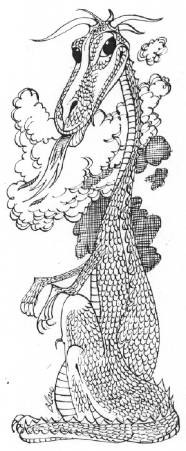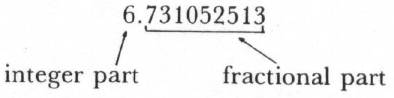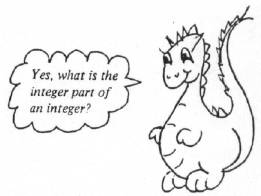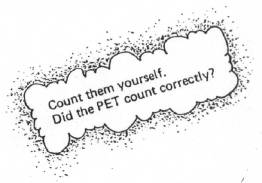`COMPUTE! ISSUE 9 / FEBRUARY 1981 / PAGE 34`

Part 2 of several

The Mysterious And Unpredictable RND

Bob Albrecht and George Firedrake

From a book of the same name by Dymax Publishing Co.; copyright © 1980 Dymax. Permission to reprint by teachers for classroom use is granted.

Integer RND Numbers

It's true. RND numbers are greater than zero and less than one.

```O < RND (1) < 1
```

Another way to say it: RND numbers are decimal fractions between 0 and 1.

But what if we want random integers from 1 to 6 (as in rolling a die) or random digits (0,1,2,3,4,5,6,7,8,9) or random integers from 1 to 100?

Well, if RND(1) is a number between 0 and 1, then 10 times RND(l) must be a number between 0 and 10. OK? Hmmm ... not so sure? Try this program.

```100 REM***RND NUMBERS BETWEEN ZERO AND TEN
110 PRINT "[CLR]";
120 INPUT "HOW MANY RND NUMBERS" ; N
130 PRINT
200 REM***PRINT N RND NUMBERS (10*RND (1))
210 FOR K = 1 TO N
220 PRINT 10 * RND (1),
230 NEXT K
240 PRINT
999 END
```

Here is a sample run.

```HOW MANY RND NUMBERS? 16
3.34464508	.328904955
3.69228884	6.31052523
7.74506208	4.35766491
5.07949568	9.26821156
9.78314249	1.95072511
8.04495845	7.15665136
.0878570662	1.47048625
7.02014795	2.49452329
▪
```

Yes, all 16 numbers are between 0 and 10. In the above sample the smallest number is .0878570662 and the largest number is 9.78314249.Now, think of each number as having an integer part to the left of the decimal point and a fractional part to the right of the decimal point.Here is a program to print random digits. Each number printed will be a single digit, 0 or 1 or 2 or 3 or 4 or 5 or 6 or 7 or 8 or 9. The random digit is computed and printed in line 220.

```100 REM***RANDOM DIGITS: 0 1 2 3 4 5 6 7 8 9
110 PRINT "[CLR]" ;
120 INPUT "HOW MANY RANDOM DIGITS" ; N
130 PRINT
200 REM***PRINT N RANDOM DIGITS
210 FOR K = 1 TO N
220 PRINT INT(10*RND(1)),
230 NEXT K
240 PRINT
999 END
```

A sample run might look like this.

```HOW MANY RANDOM DIGITS? 20
3 6 5 4
5 5 7 6
6 0 8 1
2 8 0 3
5 8 9 5
▪
```

Do you understand how the program works? The key is line 220.

It goes like this.

• RND(1) is a number between 0 and 1.
• 10*RND(1) is a number between 0 and 10.
• The integer part of 10*RND(1) is a single digit, 0 through 9.
• Aha! INT(10(RND(1)) is a single digit, 0 through 9.

Exercise 4.

1. What is the integer part of 7.15665136?_______

What is the fractional part?__________________

2. What is the integer part of 5.07949568? _________

What is the fractional part? _____________________

3. Beware! This one is tricky (but you can do it).

What is the integer part of .328904955?_______

What is the fractional part?_________________

For each RND number between 0 and 10, the integer (whole number) part is a single digit. So, let's tell the PET to keep the integer part and get rid of the fractional part.

Here's how. We will use the INT function. In case you are not already familiar with the INT function, here are some examples.

```INT(6.30152513) = 6
INT(7.15665136) = 7
INT(5.07949568) = 5
INT(.328904955) = 0
INT(1.95072511) = 1
```

For positive numbers, the INT function gives the integer part of the number and throws away the fractional part.

Exercise 5. Complete the following.

1. INT(2.49452329) = ________________________
2. INT(.0878570662) = ____________________
3. INT(7) = _______________________What happens if you ask the PET to compute the INT of a negative number? Try it and find out.

Exercise 6. Show how to rewrite line 220 to get integers in each range shown.

1. 0 or 1 220 _______________________
2. 0, 1, 2, 3, 4, or 5 220______________________
3. 0 to 99, inclusive 220 ______________________

Hmmm . . . suppose we want to simulate (imitate) rolling dice. We need integer RND numbers from 1 to 6, inclusive (1 or 2 or 3 or 4 or 5 or 6).

```220 PRINT INT(6*RND(1)) + 1,
```

Let's see now, how does that work?

```INT(6*RND(1)) is an integer, 0 to 5.
INT(6*RND(1)) + 1 is an integer, 1 to 6.
```

In our program on page 9, change line 220 as shown above. Also, change line 120, as follows.

```120 INPUT "HOW MANY DIE ROLLS" ; N
```

Go ahead — try it. Here's what happened when we did it.

```HOW MANY DIE ROLLS? 20
4 2 2 6
1 1 2 1
3 1 5 3
2 1 6 2
1 6 4 5
▪
```

Exercise 7.

Show how to rewrite line 220 to get integers in each range shown.

1. 1 or 2 220 __________________
2. 1,2,3,4,5,6,7,or 8 220 __________________
3. 1 to 100, inclusive 220 __________________
4. The next two are tricky!

5. 2 or 3 220 _________________
6. 2, 3 or 4 220 _________________

Now try these. What numbers might be printed by each PRINT statement

7. 220 PRINT INT (4*RND(1)) + 5,__________
8. 220 PRINT 2*(3*RND(1)) + 1,____________

Coin Flipper

Let's ask the PET to flip a coin for us. Well, actually let's ask the PET to simulate (imitate) flipping a coin. Here is one program to simulate flipping a coin.

```100 REM***COIN FLIPPER #1
200 REM***FIND OUT HOW MANY FLIPS
210 PRINT "[CLR]" ;
220 INPUT "HOW MANY COIN FLIPS" ; N
230 PRINT
400 REM***FLIP COIN N TIMES, PRINT EACH EVENT
410 FOR K = 1 TO N
420 COIN = INT(2*RND(1))→C will be either 0 or 1
430 IF COIN = 0 THEN PRINT "TAILS",
440 IF COIN = 1 THEN PRINT "HEADS",
450 NEXT K
460 PRINT
999 END
```

Let's try it.

```HOW MANY COIN FLIPS? 10
▪
```

We got six HEADS and four TAILS. RUN the program several times, using various numbers of flips. Count the HEADS and TAILS each time.

When we flip a coin, we expect that HEADS and TAILS are equally probable. That is, we are as likely to get HEADS as TAILS. We also expect that, if we flip a coin many times, the number of HEADS and the number of TAILS will be about the same.

Let's modify our program so that the PET counts the HEADS and TAILS. In the following program, we have added lines 300 through 320, changed lines 430 and 440, and added lines 500 and 510.

```100 REM***COIN FLIPPER #2
200 REM***FIND OUT HOW MANY FLIPS
210 PRINT "[CLR]" ;
220 INPUT "HOW MANY FLIPS" ; N
230 PRINT
300 REM***T = TAILS COUNTER H = HEADS COUNTER
310 T = 0
320 H = 0
400 REM***FLIP COIN N TIMES, COUNT TAILS AND HEADS
410 FOR K = 1 TO N
420 COIN = INT(2*RND(1))
430 IF COIN = 0 THEN PRINT 'TAILS',: T = T + 1
440 IF COIN = 1 THEN PRINT 'HEADS", : H = H + 1
450 NEXT K
500 REM***PRINT RESULTS OF N FLIPS
510 PRINT "I GOT" H "HEADS AND" T "TAILS."
999 END
```

Now a RUN will show the actual "flips" on the screen, followed by the number of HEADS and the number of TAILS.

```HOW MANY COIN FLIPS? 24
I GOT 13 HEADS AND 11 TAILS
▪
```How does the program work? In line 420, COIN will be either 0 or 1. If COIN is 0, then line 430 will cause TAILS to be printed and the value of T to be increased by 1.

```430 IF COIN = 0 THEN PRINT "TAILS", : T = T + 1
If COIN is 0, all of this is done. If COIN is not 0, none of this is done.
```

If COIN is 1, then line 440 will cause HEADS to be printed and the value of H will be increased by 1.

```440 IF COIN  =  1 THEN PRINT "HEADS",: H  = H +  1
If COIN is 1, all of this is done. If COIN is not 1, none of this is done.
```

This program is OK for small samples. However, if you ask the PET for a larger sample (for example, 1000. flips) then alas, only part of the sample will be on the screen along with the number of HEADS and the number of TAILS.

So, instead of printing HEADS or TAILS on the screen, let's tell the PET to "flip" a COIN N times and count (but don't print) the number of HEADS and the number of TAILS.

Exercise 8. Complete the following program to flip a coin N times and count the HEADS and TAILS.

```100 REM***COIN FLIPPER #3
200 REM***FIND OUT HOW MANY FLIPS
210 PRINT "[CLR]" ;
220 PRINT
230 INPUT "HOW MANY COIN FLIPS" ; N
300 REM***T = TAILS COUNTER, H = HEADS COUNTER
310 T = 0
320 H = 0
```
```400 REM***CLIP COIN N TIMES, COUNT TAILS & HEADS
410 FOR K = 1 TO N
420 COIN = INT(2*RND(1))
430 IF COIN = 0 THEN ________________
440 IF COIN = 1 THEN ________________
450 NEXT K
500 REM***PRINT RESULTS OF N FLIPS
510 PRINT "I GOT" H "HEADS AND" T "TAILS."
520 GOTO 220
```

←Go back for another bunch of flips.

A RUN might look like this.

```HOW MANY COIN FLIPS? 100
I GOT 53 HEADS AND 47 TAILS.
HOW MANY COIN FLIPS? 100
I GOT 45 HEADS AND 55 TAILS.
HOW MANY COIN FLIPS? 1000
I GOT 506 HEADS AND 494 TAILS.
HOW MANY COIN FLIPS? and so on...
```

Remember. With this program, the PET is actually simulating the coin flips, but is not printing the result of each flip. Instead, it counts the number of HEADS and the number of TAILS and, after doing the required number of flips, prints the results.

Exercise 9. Write a program to simulate flipping two coins. For a single toss, there are four possible outcomes.

```HH HT TH TT
```

We show HT and TH as different outcomes, because —

suppose we toss a nickel and a dime. The possible outcomes are like this:

 NICKEL H H T T DIME H T H T ← ← ← ← HH HT TH TT

Here is a RUN of our program to flip two coins at a time.

```HOW MANY COIN FLIPS? 20
TH TH HH TT
HH TH HT HT
TH TH TT TH
TT TT TT HH
HH HT TH HH
▪
```

Exercise 10. Instead of printing the results (HH or HT or TH or TT), count them. Write a program to flip two coins N times, then print the number of times they came up HH, HT, TH and TT. Below is a sample RUN, showing how we would like to see the results.

```HOW MANY COIN FLIPS? 1000
OUTCOME NUMBER OF TIMES
HH      243
HT      250
TH      259
TT      248